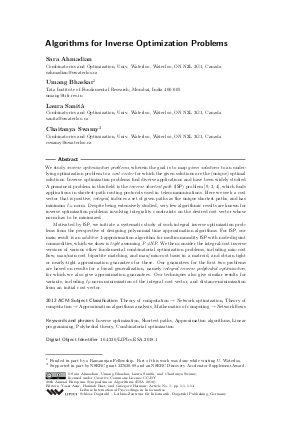Document# Algorithms for Inverse Optimization Problems## File

LIPIcs.ESA.2018.1.pdf
• Filesize: 494 kB
• 14 pages

## Cite As

Sara Ahmadian, Umang Bhaskar, Laura Sanità, and Chaitanya Swamy. Algorithms for Inverse Optimization Problems. In 26th Annual European Symposium on Algorithms (ESA 2018). Leibniz International Proceedings in Informatics (LIPIcs), Volume 112, pp. 1:1-1:14, Schloss Dagstuhl - Leibniz-Zentrum für Informatik (2018)
https://doi.org/10.4230/LIPIcs.ESA.2018.1

## Abstract

We study inverse optimization problems, wherein the goal is to map given solutions to an underlying optimization problem to a cost vector for which the given solutions are the (unique) optimal solutions. Inverse optimization problems find diverse applications and have been widely studied. A prominent problem in this field is the inverse shortest path (ISP) problem [D. Burton and Ph.L. Toint, 1992; W. Ben-Ameur and E. Gourdin, 2004; A. Bley, 2007], which finds applications in shortest-path routing protocols used in telecommunications. Here we seek a cost vector that is positive, integral, induces a set of given paths as the unique shortest paths, and has minimum l_infty norm. Despite being extensively studied, very few algorithmic results are known for inverse optimization problems involving integrality constraints on the desired cost vector whose norm has to be minimized. Motivated by ISP, we initiate a systematic study of such integral inverse optimization problems from the perspective of designing polynomial time approximation algorithms. For ISP, our main result is an additive 1-approximation algorithm for multicommodity ISP with node-disjoint commodities, which we show is tight assuming P!=NP. We then consider the integral-cost inverse versions of various other fundamental combinatorial optimization problems, including min-cost flow, max/min-cost bipartite matching, and max/min-cost basis in a matroid, and obtain tight or nearly-tight approximation guarantees for these. Our guarantees for the first two problems are based on results for a broad generalization, namely integral inverse polyhedral optimization, for which we also give approximation guarantees. Our techniques also give similar results for variants, including l_p-norm minimization of the integral cost vector, and distance-minimization from an initial cost vector.

## Subject Classification

##### ACM Subject Classification
• Theory of computation → Network optimization
• Theory of computation → Approximation algorithms analysis
• Mathematics of computing → Network flows
##### Keywords
• Inverse optimization
• Shortest paths
• Approximation algorithms
• Linear programming
• Polyhedral theory
• Combinatorial optimization

## Metrics

• Access Statistics
• Total Accesses (updated on a weekly basis)
0

## References

1. Ravindra K Ahuja, Thomas L Magnanti, and James B Orlin. Network flows: theory, algorithms, and applications. Prentice hall, 1993.2. R.K. Ahuja and J.B. Orlin. Inverse optimization. Oper. Res., 49:171-783, 2001.3. W. Ben-Ameur and E. Gourdin. Internet routing and related topology issues. SIAM J. Discrete Math., 17:18-49, 2004.4. A. Bley. Inapproximability results for the inverse shortest paths problem with integer lengths and unique shortest paths. Networks, 50:29-36, 2007.5. Endre Boros, Khaled Elbassioni, Vladimir Gurvich, and Hans Raj Tiwary. The negative cycles polyhedron and hardness of checking some polyhedral properties. Annals of Operations Research, 188(1):63-76, 2011.6. David Bremner, Komei Fukuda, and Ambros Marzetta. Primal-dual methods for vertex and facet enumeration. Discrete &Computational Geometry, 20(3):333-357, 1998.7. D. Burton, W. Pulleyblank, and Ph. L. Toint. The inverse shortest paths problem with upper bounds on shortest paths costs. In Network optimization, pages 156-171. Springer, 1996.8. D Burton and Ph L Toint. On the use of an inverse shortest paths algorithm for recovering linearly correlated costs. Mathematical Programming, 63(1):1-22, 1994.9. D. Burton and Ph.L. Toint. On an instance of the inverse shortest paths problem. Math. Program., 53:45-61, 1992.10. Michael R Bussieck and Marco E Lübbecke. The vertex set of a 01-polytope is strongly p-enumerable. Computational Geometry, 11(2):103-109, 1998.11. Mikael Call and Kaj Holmberg. Complexity of inverse shortest path routing. In INOC, pages 339-353. Springer, 2011.12. Robert B Dial. Minimal-revenue congestion pricing part i: A fast algorithm for the single-origin case. Transportation Research Part B: Methodological, 33(3):189-202, 1999.13. Robert B Dial. Minimal-revenue congestion pricing part ii: An efficient algorithm for the general case. Transportation Research Part B: Methodological, 34(8):645-665, 2000.14. Martin E Dyer. The complexity of vertex enumeration methods. Mathematics of Operations Research, 8(3):381-402, 1983.15. F. Grandoni, G. Nicosia, G. Oriolo, and L. Sanità. Stable routing under the spanning tree protocol. Operations Research Letters, 38:399-404, 2010.16. N. Haehnle, L. Sanità, and R. Zenklusen. Stable routing and unique-max coloring on trees. SIAM J. Discret. Math, 27:109-125, 2013.17. C. Heuberger. Inverse combinatorial optimization: A survey on problems, methods, and results. J. Combin. Optim., 8:329-361, 2004.18. Garud Iyengar and Wanmo Kang. Inverse conic programming with applications. Operations Research Letters, 33(3):319-330, 2005.19. Gertrud Neumann-Denzau and Jörn Behrens. Inversion of seismic data using tomographical reconstruction techniques for investigations of laterally inhomogeneous media. Geophysical Journal International, 79(1):305-315, 1984.20. J. Scott Provan. Efficient enumeration of the vertices of polyhedra associated with network LP’s. Math. Program., 63(1):47-64, 1994.21. Robert C Read. Bounds on backtrack algorithms for listing cycles, paths and spanning trees. Networks, 5:237-252, 1975.22. Alexander Schrijver. Theory of linear and integer programming. John Wiley &Sons, 1998.23. Albert Tarantola. Inverse problem theory and methods for model parameter estimation. SIAM, 2005.24. H.R. Varian. Revealed preference. Samuelsonian Economics and the Twenty-first Century, pages 99-115, 2006.25. S. Xu and J. Zhang. An inverse problem of the weighted shortest path problem. Japan J. Indust. Appl. Math., 12:47-59, 1995.26. J. Zhang and M.C. Cai. Inverse problem of minimum cuts. Mathematical Methods of Oper. Res., 47:51-58, 1998.27. J. Zhang and Z. Liu. A general model of some inverse combinatorial optimization problems and its solution method under l-infinity norm. J. Combin. Optim., 6:207-227, 2002.28. J. Zhang and Z. Ma. Solution structure of some inverse combinatorial optimization problems. J. Combin. Optim., 3:127-139, 1999.29. Jianzhong Zhang and Zhenhong Liu. Calculating some inverse linear programming problems. Journal of Computational and Applied Mathematics, 72(2):261-273, 1996.30. Jianzhong Zhang and Zhenhong Liu. A further study of inverse linear programming problems. Journal of Computational and Applied Mathematics, 106:345-359, 1999.X

Feedback for Dagstuhl Publishing